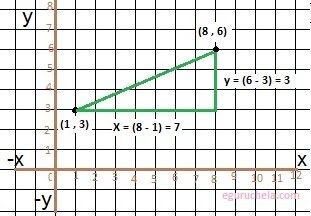# Distance between two points calculator

Find the distance between the given pairs of points.

 X1: Y1: X2: Y2 :

 Distance:

Distance Between two Points calculator calculates the distance between two given points co-ordinates (x1 , y1) and (x2 , y2) in the XY plane by applying the pythagorean theorem.

### Distance Formula

d = √ (x2 – x1)2 + (y2 - y1)2To find the distance between two points (x1,y1) and (x2,y2),
we first get the distance of x and y axis as :
x = (x2 - x1) and y = (y2 - y1).
now we apply the formula of Pythagorean theorem. $$distance = \sqrt{ x^{2} + y^{2}}$$ Let's take the example as given coordinates for two points as:
one point is (1 , 3) and second point is (8 , 6)
we will find the distance of x and y axis as follows:
$$x = (8 - 1) = 7$$ $$y = (6 - 3) = 3$$ $$distace = \sqrt {(7)^{2} + (3)^{2}}$$ $$= \sqrt {49 + 9}$$ $$= \sqrt {58}$$

• Learning